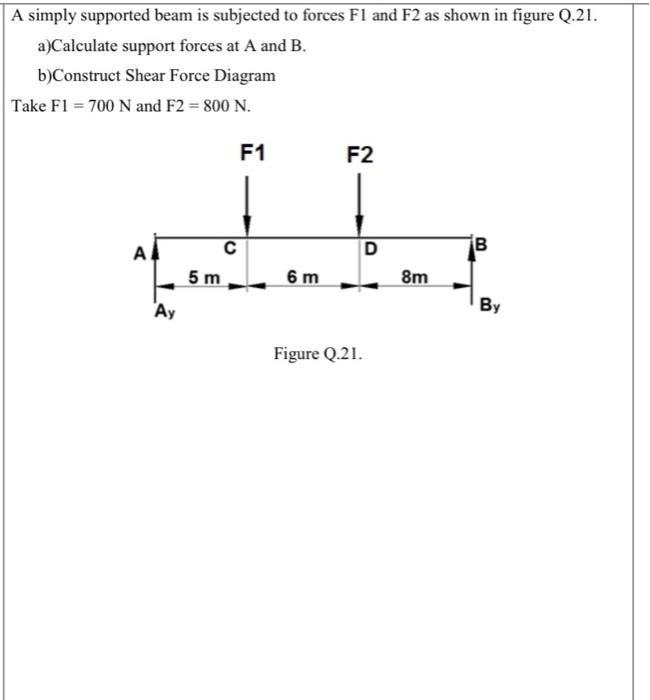### Create an Account

Home / Questions / A simply supported beam is subjected to forces F1 and F2 as shown in figure Q.21. a)Calcul...

# A simply supported beam is subjected to forces F1 and F2 as shown in figure Q.21. a)Calculate support forces at A and B. b)Construct Shear Force Diagram Take

A simply supported beam is subjected to forces F1 and F2 as shown in figure Q.21. a)Calculate support forces at A and B. b)Construct Shear Force Diagram Take F1 = 700 N and F2 = 800 N. F1 F2 Al D 00 с 5 m 6 m 8m Ay BMay 30 2021 View more View LessSubscribe To Get Solution